# What is a standard form calculator and how to use it?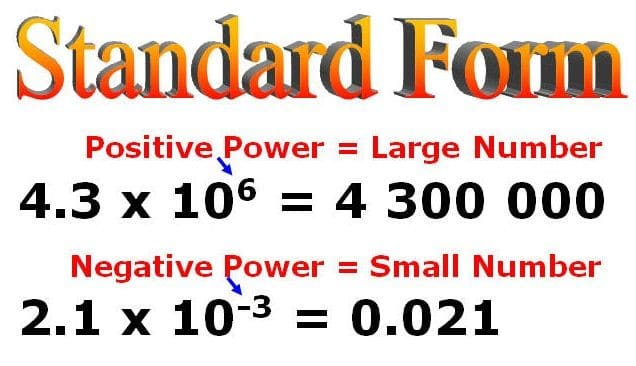So, the standard form is a mathematical device that we need widely as we go to study higher mathematics, physics, chemistry, or any such sciences. We use this to write numbers that are either very big or that are very small. So, suppose the mass of a particle is one-millionth of a gram. Now, when you are doing calculations, you cannot write the entire value- that many numbers of zeroes every time. You obviously can but that will destroy the spontaneity of the calculation. Therefore, the standard form transfers the format into 10^n. Here, n is the number of zeroes that you attach at the end of the digit. So, n can be both positive and negative. If there is multiplication, n will be positive and negative if there is division. However, this might often lead to mistakes.

So, we often need to check with a standard form calculator.However, 10^n is just the format. You can use the format in any kind of mathematical calculation with any kind of case. Therefore, in algebra, it has high importance. Squaring or tripling a value often makes things a lot easier in algebra. However, there are a number of rules with standard forms. They might be a little tedious to remember always which is why you have a standard form calculator. Moreover, you can use a standard form calculator for doing two things. You can use it to convert a big number to an exponential form. However, if you use the polynomial standard form calculator, you can easily use it for ordering the exponential forms of algebraic equations. In the sections below, we will deal with both.

### Standard form calculator online

As we already know, writing big values might be a little tricky. So, it might happen that you missed out on zero while calculating. This might in turn lead to a fatal mistake in an important calculation. Moreover, the bigger the value, the greater the difficulty. Not just that but the longer the calculation, the higher is the chance to make a mistake. Therefore, it is always better to use a standard form calculator even if not to calculate, to verify. So, on the internet, you find everything very easily. There are many standard form calculators online. Moreover, there are different kinds as well. However, we will deal with the one from calculator soup and from symbolabs.

### Standard form calculator with steps

So, let us now see how to use a standard form calculator properly with each step. Therefore, for this, we are using the standard form calculator that is available in Calculator Soup. Click on “Calculator Soup” and it will lead you to the link where you will find the calculator. So now, let us see how you will perform the operation step by step.

### Step 1 to use a standard form calculator

On opening the link you will find the standard form calculator in the shape of a rectangle on the left side. It is also called the scientific notation calculator. So now there are two panels. On the first panel, there is a search engine-like box. Therefore, here you have to enter your original value. For this case, let us say you typed 100000.

### Step 2 to use a standard form calculator

So, now you will see a clear and a calculate button just underneath the box. Therefore, check whether you have entered the value correctly and then press “calculate”. So, it will take a few seconds before coming up with the final value.

### Step 3 to use a standard form calculator

Now, in the panel below you will find the answer. So, in this case, the answer will be 10^5. Moreover, if you have any doubt or think that it is wrong you can send in your comments. So, on the answer panel of the standard form calculator, there is a “send me your comments” option. Go ahead and click it.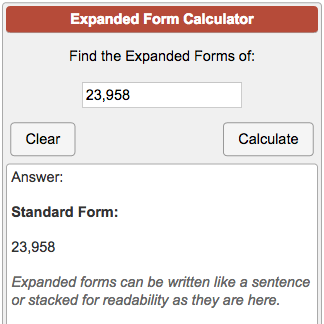### Standard form calculator fraction

So now, using standard form calculators with fractions might be a little different. This is because when there is a fraction, the exponent becomes negative. So, if I say ¼, the fractional representation is 4^-1. This is basic mathematics that we all know. Therefore, if we say one-thousandth, it means 1/1000. So, the fractional representation is 1000^-1. Or, in other terms, the standard form is 10^-3. This is because 1000 is thrice 10 or 10^3. So, 1/ 1000 must be 10^-3. Now, we often make mistakes here with the sign while doing calculations manually. Suppose you forget to put the negative sign here. So, the entire meaning of the term changes and your calculation goes drastically wrong. Therefore, to ensure that you are making no mistakes, you must use a standard form calculator in the case of fractions too.

However, the standard form calculator from calculator soup only allows scientific expressions. So, if you enter either 1/1000 or 1000^-1, it will not accept. Therefore, you have to enter the value in the form of e. e means 10. So, you enter 1e-3. -3 is the coefficient of e which in this case is negative because it is a fraction. Therefore, you get the result from the standard form calculator as 1 x 10^-3. This is your standard form of the fraction.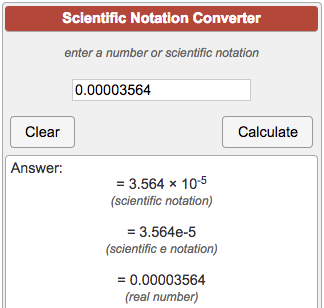### Standard form calculator rational numbers

So, as per definition, a rational number a/b is said to be in the standard form if b is positive, and the integers a and b have no common divisor other than 1. So, how does the standard form calculator work for rational numbers? There are four steps to finding this out.

Step 1: So, first get hold of the rational number.

Step 2: Verify if the denominator of the rational number is positive. In case it is negative, multiply or divide numerator and denominator both by -1. Therefore, that denominator becomes positive which is a condition for rationality.

Step 3: Now find the greatest common divisor (GCD) of the absolute values of the numerator and the denominator.

Step IV: So finally, divide the numerator and denominator of the rational number at hand by the GCD (HCF) that you found in the previous step. So, this final answer is the standard form of the given rational number.

### Standard form calculator Symbolab

Now, the standard form calculator that we find in Symbolab is a little different. It is a polynomial standard form calculator. Therefore, it does not constrict a huge number into the standard form. On the other hand, in this case, you feed in an equation, it will reorder it into the standard form. Let us understand the steps with the help of an example. Click on “Symbolab” to get the link that will direct you to the calculator.

So, on opening, you find the entire calculator. Check on the left side to see that you have selected the standard form option. Therefore, under the box containing various symbols, you will see a box in the shape of a search engine. So, inside the box, you find the word “reorder”. Hence, just beside it, enter your equation. Let us say, in this case, the equation is 2+ 4x – 5x^2. It is a quadratic equation. Now, when you have entered, click the red “go” button beside. So, it will take a few seconds to compute the result.Now, on the panel below you find the results. So, under the “solution” tab, you find the reordered polynomial. Therefore, the answer is – 5x^2 + 4x + 2. You will also find the steps below. However, you can also change the show steps setting to hide steps or step-by-step explanations. So, in the calculation, they show you the standard polynomial form and calculate in that way. Moreover, you can also verify if you have a different answer. For this, you have another search-engine-like box. Enter the answer here and click the red “Verify” button beside. So, now let us see what are the various ways in which you can use this standard form calculator.

### Standard form calculator algebra

So, every kind of polynomial is actually an algebraic expression. We have different algebra calculators on the web to simplify complex algebraic expressions. However, when we are talking about the standard form, we do not really want to solve the equation. On the other hand, we are talking about reordering the expression into the standard form by properly setting up the exponents in the hierarchy.

This is important because while doing calculations manually, it might so happen that we have a mental picture of the standard polynomial form but the coefficients we currently are handling are scattered. That might lead to very wrong and messy results. Therefore, it is always better if you reorder the polynomials in the standard form before you start to solve them. So, for this, you have the polynomial standard form calculator from symbolab. It can pretty much reorder every kind of algebraic equation that you need.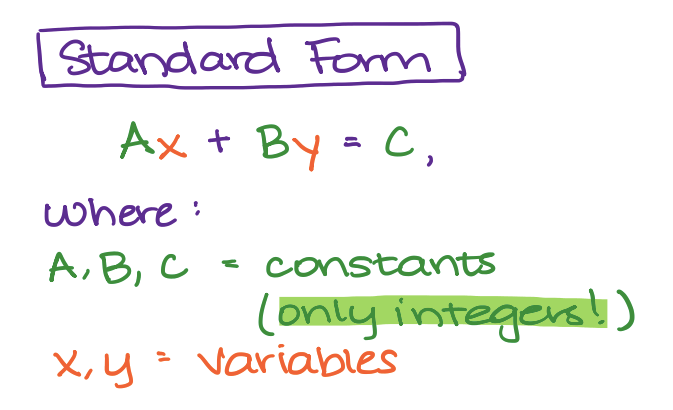Quadratic expressions are probably the polynomials that we use the most. Moreover, the first example we saw while learning the steps to use a polynomial standard form calculator was also quadratic. Let us take another example. For example, you have the expression 8x – 5 – 3x – 4x^2. Now, a quadratic equation means you cannot have a value higher than x^2.

Therefore, the standard form is ax^2 + bx + c where a, b, and c are the respective coefficients. Now, if you enter the expression in the standard form calculator the result that you will get is – 4x^2 + 5x -5. Now, there are two steps here. So, at first, there are two coefficients of x. Therefore on combining 8x and -3x, we get 5x. Now, the second step is to reorder it as per the standard polynomial form. So, the x^2 part along with the sign comes at first, and then the x, and then finally comes the constant.### Standard form calculator parabola

Now, the standard form of a parabola is a quadratic equation. So, just how the standard form calculator helps with the quadratic equations, it will be helpful for parabola equations as well. Symbolab also has a parabola calculator. So, if you enter the example above in the parabola calculator, you will find no fruitful result. Therefore, you will not find the vertices, the axis points, the foci, the intercepts, and so on. What you will find is simply that the calculator has converted the equation into its standard form. However, if you order the equation from the standard form calculator and then enter it, you will find all the appropriate results. Moreover, it will also show the step-by-step calculations. And, at any moment you can change the calculators from the left-hand-side menus.

Therefore, symbolab gives you a wide variety of options that you can access easily in the course of a calculation.### Standard form calculator slope

So, just like the parabola calculator, Symbolabs also has a slope-intercept calculator. Therefore, in the slope-intercept search box, you need to type the polynomial. And, you will find the results that you want. However, while inserting the polynomial, you will need the help of the standard form calculator again. This is because if you enter the unordered polynomial, the slope-intercept calculator will only order it instead of finding the slope. So, order and simplify the polynomial if it is a curve like a parabola or the equation of a straight line with the standard form calculator. Now, enter this ordered expression into the slope-intercept calculator. Moreover, both the calculators are available in Symbolabs. Therefore, navigating across them during calculations is very easy.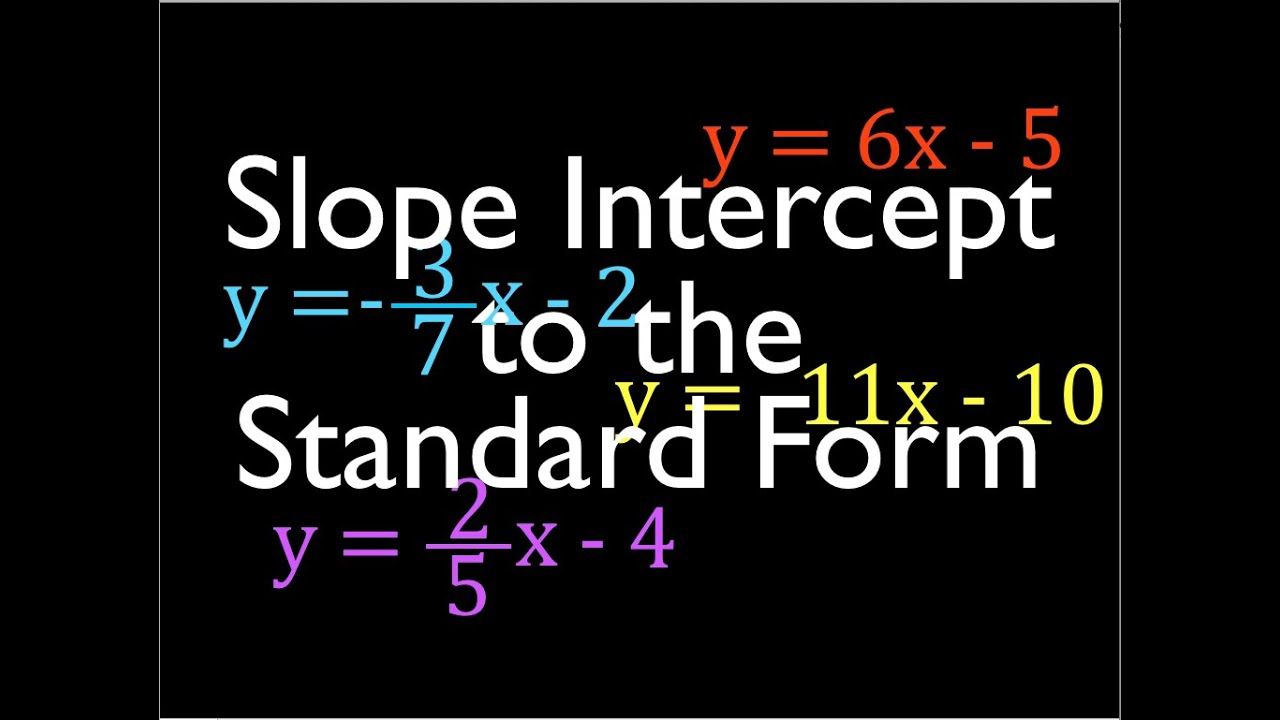### Standard form calculator FAQs

#### How do you write in standard form?

Ans. Now, the basic thing that we mean by standard form is writing huge numbers in the form of 10^n. However, you can use a standard form calculator to reorder a polynomial as well. Moreover, you can also have the standard form of a rational number which is very different from any of these. Check the sections above to know more about it.

#### How do you write 200000 in the standard form?

Ans. Well, if you follow the rule, it is very simple to understand. So, this is basically 2 x 100000. Therefore, there are 5 zeroes. So, the standard form of 200000 would be 2 x 10^5.

#### What is the standard form of 70000?

Ans. So, writing seventy thousand is definitely not its standard form. However, 70000 basically means 7 x 10000. So, the standard form is 7 x 10^4. Therefore, this is the answer that a standard form calculator will give you.

#### What is the standard form is 1000000000?

Ans. It is very important we remember that we use the standard form in terms of 10 and not 1. So, the value of the exponent will be the same as the number of zeroes that you can see. Therefore, in this case, you can either write 1 x 10^9 or 10 x 10^8. Both are correct but the standard form calculator will give you the former result.

#### What is the standard form of algebra?

Ans. So, this is a very generalized question. Various algebraic equations have various standard forms. However, the standard form of a linear equation is ax + by = c, if x and y are 2 variables. Or, it might simply be ax – c = 0. For the higher polynomials, check the standard form calculator symbolab section above.

#### What is the standard form of 3670000?

Ans. Now, as we already know, standard form always happens in the power of 10. Therefore, the standard form of 3670000 cannot be 367 x 10^4. You have to start it right from the unitary place. So, it will be 3.67 x 10^6. So, it is for these minute details that you just need a standard form calculator.

#### What is 5680219 in expanded form?

Ans. So, just how a standard form calculator simplifies a number by using the standard form, you can also simplify it by expanding. So, in this case, 5680219 cannot be brought to the standard form unless you expand. Therefore, 5680219 = 5000000 + 600000 + 80000 + 0 + 200 + 10 + 9. Now, you can find the standard forms of the individual terms. Therefore, you can have 5 x 10^6 + 6 x 10^5 + 8 x 10^4 + 0 + 2 x 10^2 + 10 + 9.

#### How do you write 0.005 in standard form?

Ans. So, this is a decimal or a fraction. Therefore the standard form will be in the form of the negative power of 10. So, the standard form of 0.005 will be 5 x 10^-3.

#### How do you write 1001 in scientific notation?

Ans. So, you always have to write the scientific notation or the standard form right from the unitary place. Hence, if you enter this value in a standard form calculator, the answer that you will get is 1.001 x 10^3.

#### Is the scientific notation of a number between 1 and 100?

Ans. Well, so the scientific notation or the standard form of a number contains 2 parts. So, this is in the form of an x 10^n. The first part of the number, which is a must be between 1 to 100. However, most standard formula calculators prefer to keep it between 1 to 10.